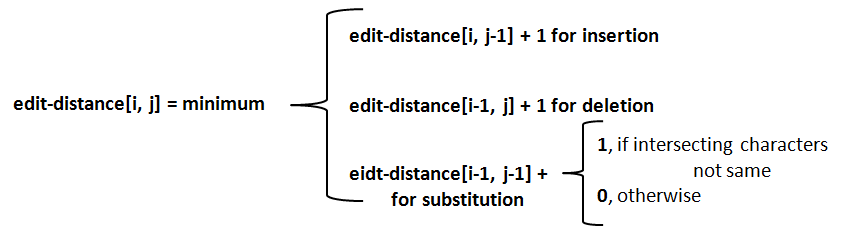## Minimum edit distance

Minimum edit distance is the minimum number of editing operations (insertion, deletion, substitution) required to convert one string into another.
It is a measure of how alike two different words are to each other.
We have two variants of minimum edit distance.
• The basic minimum edit distance where the cost for each operation is 1. That is, insertion cost = deletion cost = substitution cost = 1
• For the Levenshtein distance, the cost for insertion and deletion will be 1 2 for substitution (substitution is considered as a combination of delete and insert)
It is computed with the help of dynamic programming (Dynamic programming is the name for a class of algorithms that apply a table-driven method to solve problems. It finds the solution to the small problems and combines these solutions to find the solution for larger problem).
According to dynamic programming algorithm for word comparison, a distance matrix is created with one column for each character in the target sequence and one row for each character in the source sequence (i.e., target along the top, source along the side). Each cell in the distance matrix contains the distance between two strings. For instance, the cell intersect at i, j (distance[i, j]) contains the distance between first i characters of the target and the first j characters of the source. The value for each cell is calculated as per the equation shown below;Example:
Computation of minimum edit distance between the words DOG and COW is shown in the example below.
 # C O W # 0 1 2 3 D 1 1 2 3 O 2 2 1 2 G 3 3 2 2
Minimum edit distance is between DOG and COW is 2 which is present in the last cell in the above table.
Each cell in the above table is a minimum edit distance between a set of strings. For example, the distances between some strings are given below;
DO – CO = 1 [the cell that intersects at horizontal and vertical O holds this]
DOG – CO = 2 [the cell intersects at horizontal G and vertical O holds this]
DO – COW = 2 [the cell that intersects at horizontal O and vertical W holds this]
and so on.
Operation list converting DOG to COW is as follows;
DOG
Substitute D by n                  COG                   cost 1
Do nothing with O                COG
Substitute G by W                 COW                   cost 1
We have transformed DOG to COW with 2 edit operations.

***********
Go to NLP Glossary